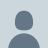Tag Archives: all basic mathematical symbols

Basic Mathematical Symbols

Mathematics: The abstract science of number, quantity, and space, either as abstract concepts (pure mathematics ), or as applied to other disciplines such as physics and engineering ( applied mathematics ). Symbol Symbol Name Meaning / definition Example = equals sign equality 5 = 2+3 ≠ not equal sign inequality 5 ≠ 4 > strict inequality greater than

more..

• Email SubscriptionLeslie99Lawrenc5Prince_Adrcathysashishpagyogynov
••••